GFG App
Open AppBrowser
Continue

# Difference between Full and Complete Binary Tree

A binary tree is a type of data structure where each node can only have two offspring at most named as “left” and “right” child.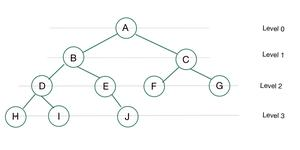A Binary Tree

There are different types of binary tree but here we are going to discuss about the difference of Complete binary tree and Full binary tree.

Full Binary Tree:

A full binary tree is a binary tree in which all of the nodes have either 0 or 2 offspring. In other terms, a full binary tree is a binary tree in which all nodes, except the leaf nodes, have two offspring.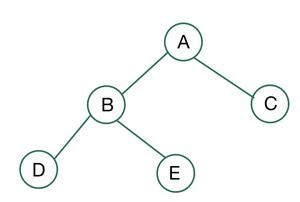A Full Binary Tree

Let, i be the number of internal nodes
n be the  total number of nodes
l be number of leaves
λ be number of levels

Then,

The number of leaves is (i + 1).
The total number of nodes is (2i + 1).
The number of internal nodes is (n – 1) / 2.
The number of leaves is (n + 1) / 2.
The total number of nodes is (2l – 1).
The number of internal nodes is (l – 1).
The number of leaves is at most (2λ – 1).

Complete Binary Tree:

A binary tree is said to be a complete binary tree if all its levels, except possibly the last level, have the maximum number of possible nodes, and all the nodes in the last level appear as far left as possible.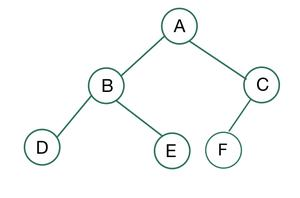A Complete Binary Tree

There are 2 points that you can recognize from here,

1. The leftmost side of the leaf node must always be filled first.
2. It isn’t necessary for the last leaf node to have a right sibling.

Check the following examples to understand the full and complete binary tree in a better way.

Example 1: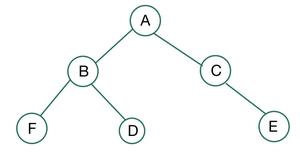Neither complete nor full

• Node C has just one child therefore, it is not a Full binary tree.
• Node C also has a right child but no left child, therefore it is also not a Complete binary tree.

Hence, the binary tree shown above is neither complete nor full binary tree.

Example 2: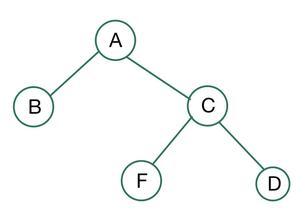Full but not complete

• All of the nodes have either 0 or 2 offspring, therefore, it is a Full binary tree
• It is not a Complete binary tree because node B has no children whereas node C has children, and according to a complete binary tree, nodes should be filled from the left side.

Hence, the binary tree shown above is a Full binary tree and it is not a Complete binary tree.

Example 3: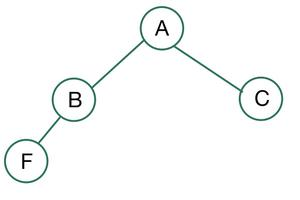Complete but not full

• It is a complete binary tree as all the nodes are left filled.
• Node B has just one child, therefore, it is not a full binary tree.

Hence, the binary tree shown above is a Complete binary tree and it is not a Full binary tree.

Example 4: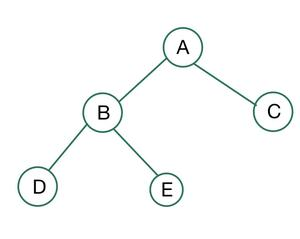Complete and full

• It is a Complete binary tree because all the nodes are left filled.
• All of the nodes have either 0 or 2 offspring, therefore, it is a full binary tree.

Hence, the binary tree shown above is both a complete and a full binary tree.

My Personal Notes arrow_drop_up